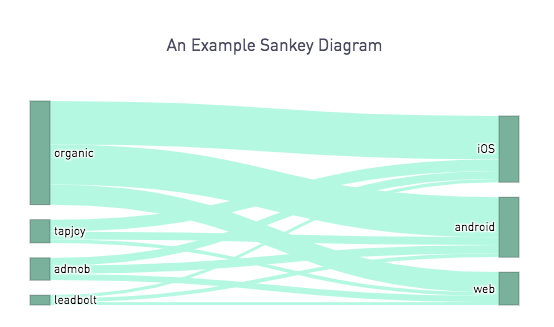# Sankey Diagrams with Plot.ly in PeriscopeSankey diagrams are a very easy-to-digest way to look at the flow of objects between different points. The example we will walk through here is a basic Sankey overview, leveraging data from a fictional gaming company. The emphasis of this post is focused on how to get your pandas dataframe data into something that can be used by plot.ly in a Sankey diagram. The Sankey set up itself is modeled after the first example in the documentation here

If you want to look at different Sankey plots from a visualization standpoint, plot.ly has a great library or resources. I've linked some of those resources at the bottom of this post for further reference

Without any further ado, here is the annotated code! Note that you would need the Periscope Python and R integration to use our Plot.ly integration.

Our SQL output is a dataframe that contains 3 columns:

• Source - The source that the user came from
• Platform - TThe user's platform of choice
• Count - The number of users for that source-platform combination

The SQL to generate this is noted below for reference

```select
source
, platform
, count(*)
from
users
group by
1
, 2```

And here is the Python 3.6 code, annotated up top where we set up our pandas dataframe df for use in plot.ly.

```# SQL output is imported as a dataframe variable called 'df'
import pandas as pd
import plotly.plotly as py

# First, we get a list of all of sources, remove duplicates, and make this a list
sources = df["source"].drop_duplicates().tolist()

# Then, we get a list of all of platforms (our targets), remove duplicates, and make this a list
platforms = df["platform"].drop_duplicates().tolist()

# Finally, create a list of all our nodes. We will use this for giving an id to each node for plot.ly
all_nodes = sources + platforms

# Keeping the size of our dataframes, this would be useful for applying the same color for each "node" and "link" of our sankey diagram, if we so choose to do so
n = len(all_nodes)
n2 = len(df["source"])

# Create a dataframe that has all of the node ids. We will join this to the original data frame to reference later
df1 = pd.DataFrame(all_nodes, columns = ['node'])
df1 = df1.reset_index()
df2 = pd.merge(pd.merge(df, df1, how = 'inner', left_on = "source", right_on ="node"), df1, how = 'inner', left_on = "platform", right_on ="node", suffixes = ('_source','_target'))

# Setting up the data in the plotly "data" argument.
# The nodes are described in the "node" dictionary (these are the vertical rectangles in the diagram)
# The links are described in the "link" dictionary. These have 3 attributes, the "source" (the index of the node they start at), the "target" (the index of the node they end at), and the "value" the thickness of the band. Additional attributes, such as color can also be specified.
data = dict(
type='sankey',
node = dict(
thickness = 20,
line = dict(
color = "#435951",
width = 0.5
),
label = all_nodes,
color = ["#84baa6"] * n
),
source = df2["index_source"],
target = df2["index_target"],
value = df2["count"],
color = ['#bdf9e5'] * n2
))

# Setting up the layout settings in the "layout" argument
layout =  dict(
title = "An Example Sankey Diagram",
font = dict(
size = 12
)
)

fig = dict(data=[data], layout=layout)

# Use Periscope to visualize a dataframe, text, or an image by passing data to periscope.table(), periscope.text(), or periscope.image() respectively.
periscope.plotly(fig)
```